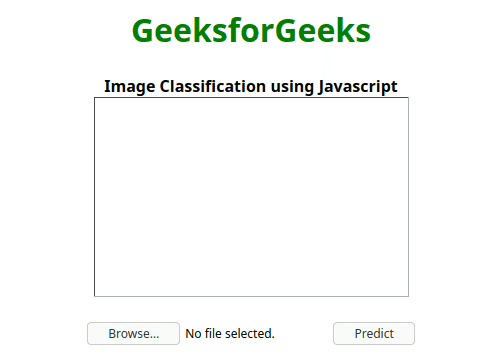# Image Classification using JavaScript

Image Classification is one of the most common applications of machine learning. Image classification is a computer vision technique in which we classify images according to the visual content in it. The example we can train an image classifier that can predict if a given image is a dog or not. In this article we would use ml5 js which is a machine learning library for the web.

Transfer Learning: In machine learning, it is a very popular technique. In this technique, we use a machine learning model trained for one task, used for another similar task. Here we would use Mobile Net a pre-trained machine learning model for image classification tasks.

• HTML Code: Here, we have created a html file and used ml5 js cdn to use it in our file. We have created an input field to upload image files. A onchange event listener is set to loadFile() function which we will define in a separate javascript file. Also, a blank image field is added to show the uploaded image.
index.html:

 `<``html` `lang``=``"en"``> ` `    ``<``head``> ` `        ``<``meta` `charset``=``"UTF-8"` `/> ` `        ``<``meta` `name``=``"viewport"`  `              ``content="``width``=``device``-width, ` `                       ``initial-scale``=``1``.0" /> ` `        ``<``title``>Image Classifier using ML5 js ` `        ``<``script` `src``= ` `"https://unpkg.com/ml5@0.4.3/dist/ml5.min.js"``> ` `        `` ` `    `` ` `    ``<``body``> ` `        ``<``center``> ` `            ``<``h1` `style``=``"color: green;"``>GeeksforGeeks ` `            ``<``b``> ` `                ``Image Classification using Javascript ` `            `` ` `            `` ` `            ``<``img` `src``=``""` `alt``=``""` `id``=``"image"`  `                 ``width``=``"315px"` `height``=``"200px"` `/> ` `            `` ` `            ``<``input` `type``=``"file"` `accept``=``"image/*"`  `                   ``onchange``=``"loadFile(event)"`  `                   ``name``=``"image"` `id``=``"file"` `/> ` `            ``<``button` `onclick``=``"predict()"``>Predict ` `        `` ` `    `` ` ` `

• JS Code: Create a loadFile function in script.js file if you prefer external js file. Firstly the image is fetched from the input field from the HTML file and the image is set inside the blank image field. Now we would initiate our machine learning model. Then ml5.imageClassifier method is called an argument ‘MobileNet’ is passed so that MobileNet is loaded for transfer learning and the second parameter is a callback function which logs ‘Model Loaded !’ in the console when the model is loaded. Now create a predict function in js section and the classifier.predict method is called. The first argument is the image that has to be classified and the second argument is a callback function. Now we use the alert method to show the predicted result of the model. This function will be called when the predict button is clicked.

 ` `

Final Solution: In this section we will just combine the above two code section into one to achieve the the

 `<``html` `lang``=``"en"``> ` `    ``<``head``> ` `        ``<``meta` `charset``=``"UTF-8"` `/> ` `        ``<``meta` `name``=``"viewport"`  `              ``content``=``"width=device-width, initial-scale=1.0"` `/> ` `        ``<``title``>Image Classifier using ML5 js ` `        ``<``script` `src``= ` `"https://unpkg.com/ml5@0.4.3/dist/ml5.min.js"``> ` `        `` ` `     ``<``script``> ` `        ``var loadFile = function (event) { ` `        ``var image = document.getElementById("image"); ` `        ``image.src = URL.createObjectURL(event.target.files); ` `       ``}; ` `       ``const classifier = ml5.imageClassifier ` `       ``("MobileNet", modelLoaded); ` ` `  `       ``// When the model is loaded ` `       ``function modelLoaded() { ` `       ``console.log("Model Loaded!"); ` `       ``} ` `             `  `       ``function predict() { ` `       ``classifier.predict(document.getElementById("image"),  ` `              ``function (err, results) { ` `                    ``alert(results.label); ` `                ``}); ` `            ``} ` ` `  `        `` ` `    `` ` `    ``<``body``> ` `        ``<``center``> ` `            ``<``h1` `style``=``"color: green;"``>GeeksforGeeks ` `            ``<``b``> ` `                ``Image Classification using Javascript ` `            `` ` `            `` ` `            ``<``img` `src``=``""` `alt``=``""` `id``=``"image"`  `                 ``width``=``"315px"` `height``=``"200px"` `/> ` `            `` ` `            ``<``input` `type``=``"file"` `accept``=``"image/*"`  `                   ``onchange``=``"loadFile(event)"`  `                   ``name``=``"image"` `id``=``"file"` `/> ` `            ``<``button` `onclick``=``"predict()"``>Predict ` `        `` ` `    `` ` ` `

Output:My Personal Notes arrow_drop_upCheck out this Author's contributed articles.

If you like GeeksforGeeks and would like to contribute, you can also write an article using contribute.geeksforgeeks.org or mail your article to contribute@geeksforgeeks.org. See your article appearing on the GeeksforGeeks main page and help other Geeks.

Please Improve this article if you find anything incorrect by clicking on the "Improve Article" button below.